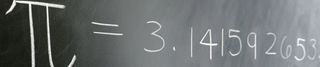# Why Pi Matters

What was so special about 9:26:53 am today? Well, the date is 3/14/15 and at that time, the date and time together would have been the same as the first ten digits of Pi: 3.141592653. It is also Albert Einstein's birthday today. Here's Steven Strogatz in The New Yorker:Pi does deserve a celebration, but for reasons that are rarely mentioned. In high school, we all learned that pi is about circles. Pi is the ratio of a circle’s circumference (the distance around the circle, represented by the letter C) to its diameter (the distance across the circle at its widest point, represented by the letter d). That ratio, which is about 3.14, also appears in the formula for the area inside the circle, A = πr2, where π is the Greek letter “pi” and r is the circle’s radius (the distance from center to rim). We memorized these and similar formulas for the S.A.T.s and then never again used them, unless we happened to go into a technical field, or until our own kids took geometry.

So it’s fair to ask: Why do mathematicians care so much about pi? Is it some kind of weird circle fixation? Hardly. The beauty of pi, in part, is that it puts infinity within reach. Even young children get this. The digits of pi never end and never show a pattern. They go on forever, seemingly at random—except that they can’t possibly be random, because they embody the order inherent in a perfect circle. This tension between order and randomness is one of the most tantalizing aspects of pi.

Pi touches infinity in other ways. For example, there are astonishing formulas in which an endless procession of smaller and smaller numbers adds up to pi. One of the earliest such infinite series to be discovered says that pi equals four times the sum 1 – + – + – + ⋯. The appearance of this formula alone is cause for celebration. It connects all odd numbers to pi, thereby also linking number theory to circles and geometry. In this way, pi joins two seemingly separate mathematical universes, like a cosmic wormhole.

But there’s still more to pi.

More here.